Next: Heavy artillery Up: GEOMETRY-BASED DECON Previous: Regressing simultaneously before and

## A model for convolution both before and after NMO

Here we will develop a formal theory for (1). By formalizing the theory, we will see better how it can be made more precise, and how the wishes expressed by (1) are a linearization of a nonlinear theory.

For a formal model, we will need definitions. Simple multiple reflections are generated by 1/(1+cZn), where c is a reflection coefficient and Zn is the two-way traveltime to the water bottom. We will express reflectivity as an unspecified filter R(Z), so the reverberation operator as a whole is 1/(1+R(Z)), where R(Z) is like the adjustable coefficients in a gapped filter. This form is partly motivated by the idea that 1>|R|. Taking xt to denote the reflection coefficients versus depth or the multiple-free seismogram, and taking yt to denote the one-dimensional seismogram with multiples, we find that the relation between them is conveniently expressed with Z-transforms as Y(Z)=X(Z)/(1+R(Z)).

Likewise, we will express the source signature not as a convolution but as an inverse polynomial (so designature turns into convolution). Suppose that source signature as a whole is given by the operator 1/(1+S(Z)). The final data D(Z) is related to the impulse-source seismogram Y(Z) by D(Z)=Y(Z)/(1+S(Z)).

The trouble with the definitions above is that they are in the Fourier domain. Since we are planning to mix in the NMO operator, which stretches the time axis, we will need to reexpress everything in the time domain. Instead of X(Z)=Y(Z)(1+R(Z)) and Y(Z)=D(Z)(1+S(Z)), we will use shifted-column matrices to denote convolution. Thus our two convolutions can be written as(2) (3)
whereis an identity matrix. Combining these two, we have a transformation from the data to the reflection coefficients for a one-dimensional seismogram. Departures from one-dimensionality arise from NMO and from spherical divergence

of amplitude. Simple theory (energy distributed on the area of an expanding sphere) suggests that the scaling factor t converts the amplitude ofto.So we define a matrixto be a diagonal with the weight t distributed along it.

We need also to include the time shifts of NMO. In chapterwe saw that NMO is a matrix in which the diagonal line is changed to a hyperbola. Denote this matrix by.Let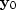be the result of attempting to generate a zero-offset signal from a signal at any other offset by correcting for divergence and moveout:(4)

The NMO operator can be interpreted in two ways, depending on whether we plan to find one filter for all offsets, or one for each. In other words, we can decide if we want one set of earth reflection coefficients applicable to all offsets, or if we want a separate reflection coefficient at each offset. From chapterwe recall that the more central question is whether to include summation over offset in the NMO operator. If we choose to include summation, then the adjoint sprays the same one-dimensional seismogram out to each offset at the required moveout. This choice determines if we have one filter for each offset, or if we use the same filter at all offsets.

Equation (2) actually refers only to zero offset. Thus it means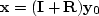.Merging this with equations (3) and (4) gives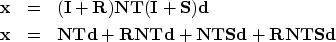(5) (6)

Now it is time to think about what is known and what is unknown. The unknowns will be the reverberation operators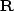and.Since we can only solve nonlinear problems by iteration, we linearize by dropping the term that is the product of unknowns, namely, the last term in (6). This is justified if the unknowns are small, and they might be small, since they are predictions. Otherwise, we must iterate, which is the usual solution to a nonlinear problem by a sequence of linearizations. The linearization is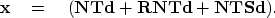(7)
When a product of Z-transforms is expressed with a shifted-column matrix, we have a choice of which factor to put in the matrix and which in the vector. The unknown belongs in the vector so that simultaneous equations can be the end result. We need, therefore, to rearrange the capital and lower-case letters in (7) to place all unknowns in vectors. Also, besides the original data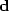,we will be regressing on processed data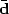,defined by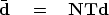(8)
Equation (7) thus becomes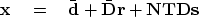(9)
Now the unknowns are vectors.

Recall that the unknowns are like prediction filters. Everything inthat is predictable byandis predicted in an effort to minimize the power in.During the process we can expectto tend to whiteness. Thus our statement of wishes is(10)
Equation (10) is about the same as (1). To see this, associatewith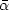and associatewith.To make (10) look more like a familiar overdetermined system, I write it as(11)

Some years ago I tested this concept on a small selection of data, including Yilmaz and Cumro dataset 27, used in Figure 6. The signature waveform of this dataset was hardly measurable, and almost everything was in the reverberation. Thus, results nearly equal to Figure 6 could be obtained by omitting the deconvolution before NMO. Although I was unable to establish by field-data trials that simultaneous deconvolution is necessary, I feel that theory and synthetic studies would show that it is.Next: Heavy artillery Up: GEOMETRY-BASED DECON Previous: Regressing simultaneously before and
Stanford Exploration Project
10/21/1998